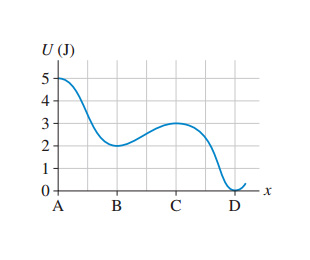# Problem: The following image is the potential-energy diagram for a 500 g particle that is released from rest at A.(a) What is the particles speed at B?(b) What is the particles speed at C?(c) What is the particles speed at D?

🤓 Based on our data, we think this question is relevant for Professor Thompson's class at MC MARICOPA.

###### Problem Details

The following image is the potential-energy diagram for a 500 g particle that is released from rest at A.
(a) What is the particles speed at B?
(b) What is the particles speed at C?
(c) What is the particles speed at D?